# Projective transformation

A one-to-one mappingof a projective space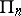onto itself preserving the order relation in the partially ordered (by inclusion) set of all subspaces of, that is, a mapping ofonto itself such that:
1) if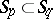, then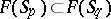;
2) for every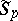there is an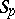such that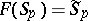;
3)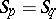if and only if.
Let the spacebe interpreted as the collection of subspacesof the left vector space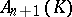over a skew-field. A semi-linear transformation of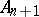into itself is a pair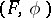consisting of an automorphismof the additive groupand an automorphismof the skew-fieldsuch that for any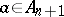and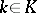the equality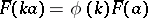holds. In particular, a semi-linear transformationis linear if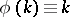. A semi-linear transformationinduces a projective transformation. The converse assertion is the first fundamental theorem of projective geometry: If, then every projective transformationis induced by some semi-linear transformationof the space.